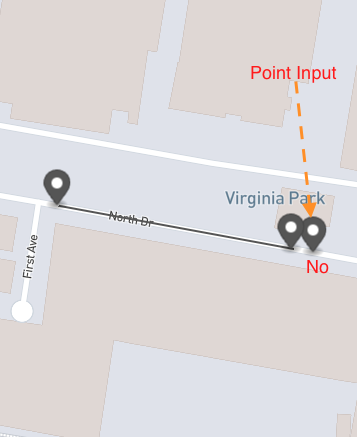# How to determine if a point given is within a line (two points)

I have got a line with two points and a single point. I need to check whether the single point is between the line (i.e. between the two points) given, including the two points given.

How do I do that using Julia.``````line_string = [
[
145.06226370532733,
-37.90964817711629
],
[
145.06220165065736,
-37.90997457594736
]
]

point = [145.06220165065736, -37.90997457594736]
``````

One solution is:

``````using GeoStats

Point(0.5, 0.5) ∈ Segment((0.0, 0.0), (1.0, 1.0))
``````

Check chapter 4 of the book:

https://juliaearth.github.io/geospatial-data-science-with-julia/

3 Likes

Check if the normalized dot product of the line with the vector from one end of the line to the point is between zero and one:

``````julia> using LinearAlgebra
julia> between(a, b, p) = 0 <= dot((b - a) / norm(b - a), (p - a) / norm(b - a)) <= 1
between (generic function with 1 method)

julia> between(line_string, line_string, point)
true
``````
1 Like

This does not seem 100% right.

``````a=[0.0,0.0]
2-element Vector{Float64}:
0.00000e+00
0.00000e+00

julia> b=[1.0,0.0]
2-element Vector{Float64}:
1.00000e+00
0.00000e+00

julia> p=[.5,.5]
2-element Vector{Float64}:
5.00000e-01
5.00000e-01

julia> between(a,b,p)
true
``````

but (1/2, 1/2) is not between (0,0) and (1,0)

I think we are a bit confused by your question.

1. Do you mean whether the point is lying on the line segment between two points? (Then your initial question should be answered no, as that point is slightly off the line)
2. Do you mean the point is in the “slice” of plane between the two points (as defines by the normal to the line segment connecting the two points, at those two points)? In that case, the latter question should be yes.
1 Like

O also got confused with te quedtion. if question is 1. then compute distante to the line and see if it is smaller than an eps of your choice.

Yupp, it doesn’t check if the point is actually on the line. For that, you need to check if the norm of the cross product is (near) zero. My snippet above checks if the point is between the two endpoints assuming it is on the line.

Here’s an adjusted version that also checks for being on the segment:

``````function between(a, b, p; tol = 1e-10)::Bool
v1 = b - a
v2 = p - a
return isapprox(dot(v1, v2), norm(v1) * norm(v2); rtol = tol) &&
-tol <= dot(v1 / norm(v1), v2 / norm(v1)) <= 1 + tol
end
``````
2 Likes

All this confusion is one of my main gripes with GIS standards. Back in the days, someone decided to give the name “line” to what actually is a line segment in mathematics.

@Chamika_Kasun you can always check the source code of our Meshes.jl submodule when you need to learn about these geometric algorithms, it is plain simple Julia code:

Don’t reinvent the wheel re-implementing these algorithms from scratch, because they can be quite tricky to get right. There are too many corner cases that we are still fixing with various industrial applications.

1 Like

“line” and “between” have been defined for 1000s of years. I don’t see any serious opportunity for confusion or misunderstanding here.

Here’s an example “I’m a liberal somewhere between Trump and DeSantis and I’m not line.”

The confusion exists because GIS Line and LineString should have been called LineSegment and polygonal Chain.

A line is infinite, a segment is not.

2 Likes

I recal that data in OP’s example is geographical, so none e of the above solutions do really apply.

At the scale of the screenshots, the solution will apply.

Algorithms for geographic coordinates will be added in the near future. It is an implementation detail that we also plan to hide from end users, who don’t care about all this GIS idiosyncrasy.

1 Like

Hence OP example in the question is wrong, as that point is NOT on the line.Or, maybe, OP is intending those terms in a slight different way from the geometrical one. As you would when you say that Florence is between Milan and Rome.

Thus, there is some confusion